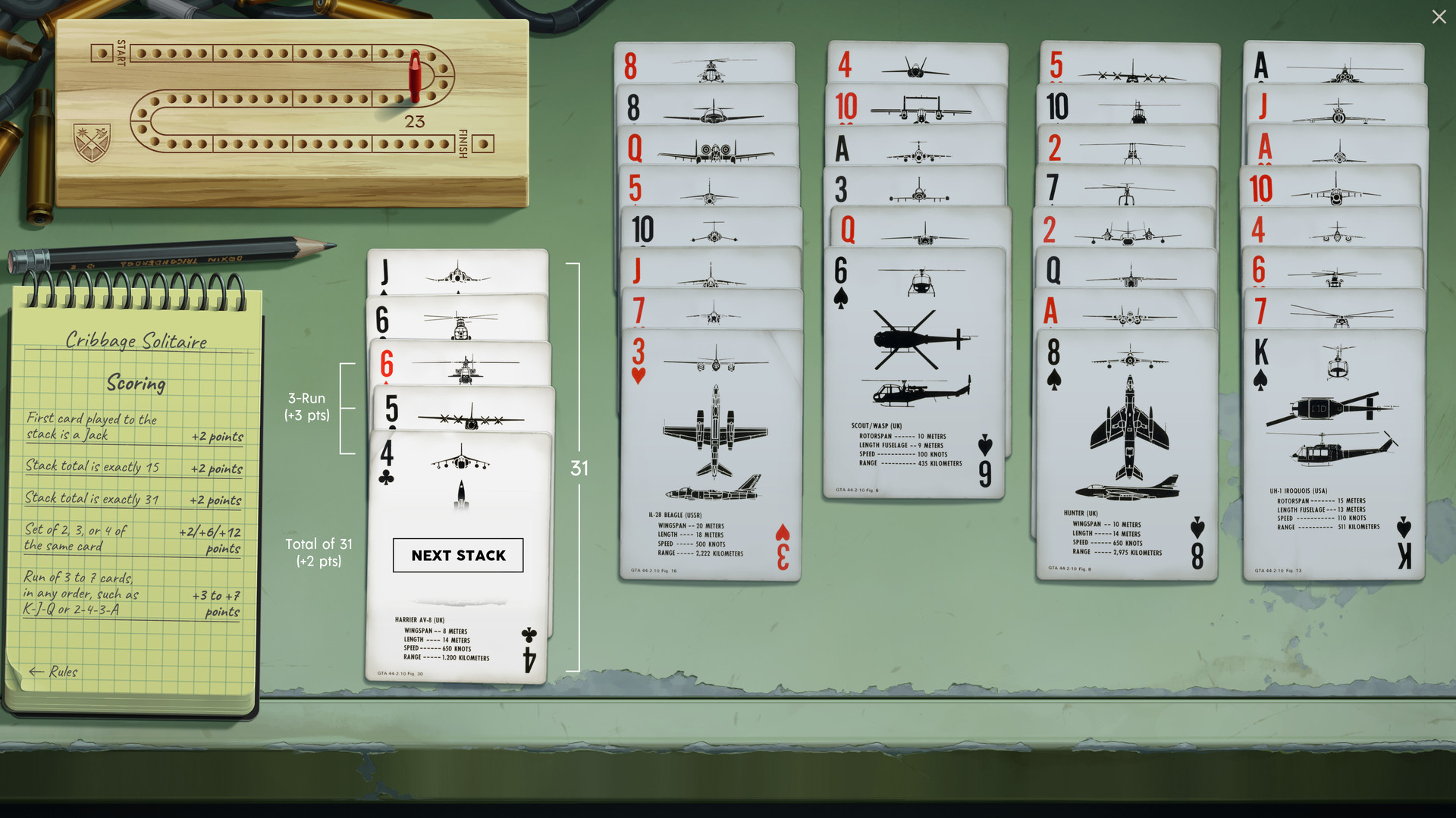Zachtronics’s recent game Möbius Front ‘83 – like most of their offerings – contains an optional card game inside. This time it’s a solitaire version of cribbage, where you get points for playing cards in sets and combos. It rewards careful thinking and planning ahead, which sounds like a lot of work; let’s get the computer to do it instead.You start with a standard deck of 52 cards dealt in 4 piles. You can play the top card of any pile onto a stack, but the stack’s total value can’t go over 31. You get points when the stack meets certain conditions: if the stack’s value is exactly 15 or 31, or if you play 2/3/4 of a kind in a row, or if the last few cards in the stack can be arranged into a run of consecutive values. When you can’t play any more cards without exceeding 31, you throw the stack away and start with a fresh, empty stack. The aim is to get as many points as possible.

One approach to an automatic solver for the game is a greedy one: look at the position you’re in, find the best possible “stack” of moves, and commit to it. Then continue doing this until all the cards are played. In my experiments this proved not very good, usually failing to reach the game’s target score of 61 points. The problem is that making the “best” move right now might prevent us from scoring even more points later on.

A simple modification of this approach is to introduce some randomness: instead of always choosing the highest-scoring move, choose a “good” move at random. With a bit of randomness, we can repeatedly run the algorithm in the hope of chancing upon a high-scoring combination of moves. This modification can be done by putting all the moves in order (highest-scoring first), then walking along them and flipping a weighted coin. If the coin comes up heads, commit to that move; otherwise, continue to the next move.

Roughly speaking, the algorithm is:

void probe(GameState state) {
if all cards are played
if this is the best score, print it out and remember it
else
compute all the possible moves
pick a good one and execute it
probe(new state)
}


This approach in practice gives good-enough solutions very fast. But if we want really big scores, we can use a complete algorithm that will always find the best possible score.

A key property of the game is that what you did before doesn’t influence what you should do next. That is, if I play the first half of the game and then you take over, you don’t need to know exactly what I did or even how many points I scored – you only need to know which cards are left for you to play. This property suggests a dynamic programming approach.

Dynamic programming as applied to this game works as follows. From a given position, we can calculate the best total score we can possibly get. Let’s say we are in position $$P$$ and we’re considering move $$M$$. Move $$M$$ will gain us $$S_M$$ score immediately, and leave us in a new position $$P_M$$. The function $$f(P)$$ gives the best score attainable from position $$P$$, and is defined as:

$f(P) = \max(\{ S_M + f(P_M) \mid M \in \mathit{moves}(P)\})$

That is, the best score is via whichever move gives the highest sum of “points gained from that move” and “points available from the position we’re in after the move”.

We can keep the definition of “position” simple by assuming that the current stack is always empty. That means each move must be a whole stack’s worth of play. Then a position can be described by just four numbers: how many cards have we used up from each pile so far? This position is the key into a cache where we record the answers so they can be reused when we inevitably encounter the same sub-positions again.

So the algorithm is:

Score get(Position p) {
if p is the end position, return 0
if p is in the cache, return the cached score
compute all the moves from p
find the one that maximises score(move) + get(position-after-move)
store that maximum score in cache
return that score
}


According to some very non-rigorous tests, my implementation of this approach takes about 30 seconds to find the optimal solution. Some basic profiling suggests the slowest part is in the scoring of runs of consecutive digits, where there is definitely opportunity for improvement.

Both methods are implemented in Java in this GitHub repository. The first method is in the probe function in Search.java, and the dynamic programming method is in the get function in Dynamic.java.